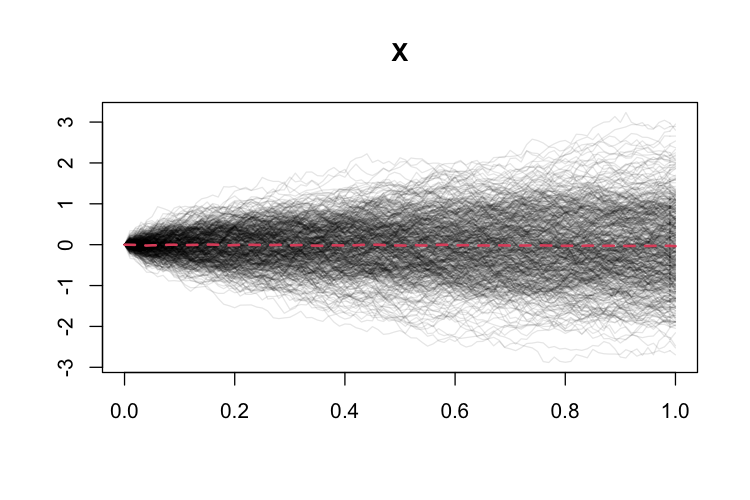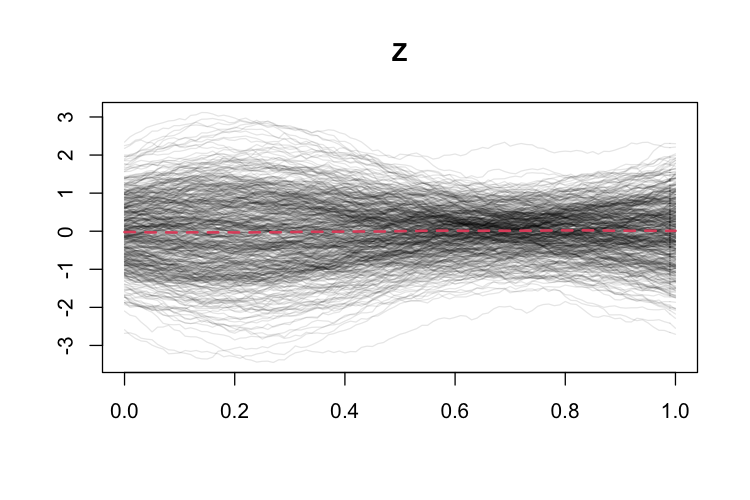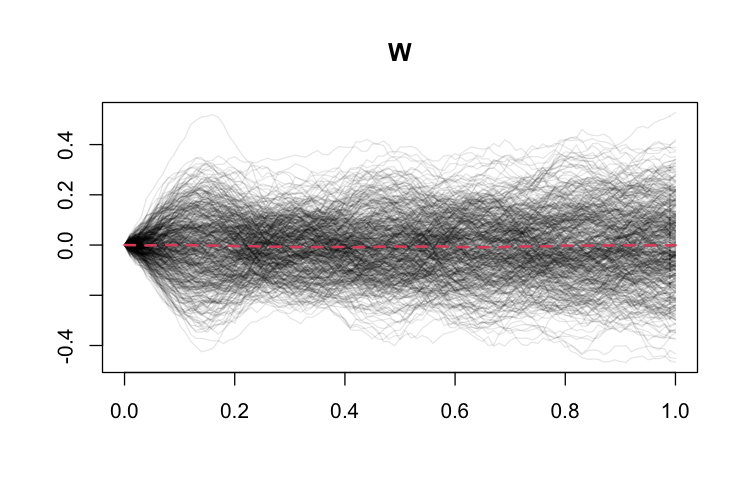# Introduction

ghcm is an R package used to perform conditional independence tests for functional data.

This vignette gives a brief overview of the usage of the ghcm package. We give a brief presentation of the idea behind the GHCM and the conditions under which the test is valid. Subsequently, we provide several examples of the usage of the ghcm package by analysing a simulated data set.

# The Generalised Hilbertian Covariance Measure (GHCM)

In this section we briefly describe the idea behind the GHCM. For the full technical details and theoretical results, see .

Let $$X$$, $$Y$$ and $$Z$$ be random variables of which we are given $$n$$ i.i.d. observations $$(X_1, Y_1, Z_1), \dots, (X_n, Y_n, Z_n)$$; here $$X$$, $$Y$$ and $$Z$$ can be either scalar or functional. Existing methods, such as the GCM  implemented in the GeneralisedCovarianceMeasure package , can deal with most cases where both $$X$$ and $$Y$$ are scalar hence our primary interest is in the cases where at least one of $$X$$ and $$Y$$ are functional.

The GHCM estimates the squared Hilbert–Schmidt norm of the expected conditional covariance of $$X$$ and $$Y$$ given $$Z$$, $$\| \mathscr{K} \|_{\mathrm{HS}}^2$$, and rejects the hypothesis $$X \mbox{{}\perp\mkern-11mu\perp{}}Y \,|\,Z$$ if this quantity is too large. We denote by $$\langle f, g \rangle$$ the inner product between $$f$$ and $$g$$. We compute the GHCM as follows.

1. Regress $$X$$ on $$Z$$ and $$Y$$ on $$Z$$ yielding residuals $$\hat{\varepsilon}$$ and $$\hat{\xi}$$, respectively.
2. Compute the test statistic $T = \frac{1}{n} \sum_{i=1}^n \sum_{j=1}^n \langle \hat{\varepsilon}_i, \hat{\varepsilon}_j \rangle \langle \hat{\xi}_i, \hat{\xi}_j \rangle.$
3. Compute the $$n \times n$$ matrix $$\Gamma$$ by setting $\Gamma_{ij} := \langle \hat{\varepsilon}_i, \hat{\varepsilon}_j \rangle \langle \hat{\xi}_i, \hat{\xi}_j \rangle.$
4. Compute the $$d \leq n-1$$ non-zero eigenvalues $$(\lambda_k)_{k=1}^d$$ of $C := \frac{1}{n-1} (\Gamma - J \Gamma - \Gamma J + J \Gamma J),$ where $$J$$ is the $$n \times n$$ matrix with all entries equal to $$1/n$$.
5. Produce a $$p$$-value by setting $p := \mathbb{P}\left(\sum_{k=1}^d \lambda_k \zeta_k^2 > T\right),$ where $$\zeta_1, \dots, \zeta_d$$ are i.i.d. standard Gaussian random variables.

Assuming that the regression methods perform sufficiently well, the GHCM has approximately uniformly distributed $$p$$-values when the null is true. It should be noted that there are situations where $$X \mbox{{}\not\!\perp\mkern-11mu\perp{}}Y \,|\,Z$$ but the GHCM is unable to detect this dependence for any sample size, since $$\mathscr{K}$$ can be zero in this case.

The GHCM as implemented in the function uses different methods to compute the inner products as required above depending on the format of the given residuals. For residuals from scalar or multivariate variables or functional variables observed on a constant, fixed grid, the inner products are computed as the usual Euclidean inner products and no further preprocessing is done. For residuals coming from irregularly observed functional variables or functions on a fixed grid with missing values, the inner products are computed as L2 inner products computed from spline interpolants of the curves. See the following sections for examples of both types of use.

# Example applications on a simulated dataset

## The GHCM with regular functional data

To give concrete examples of the usage of the package, we perform conditional independence tests on a simulated data set consisting of both functional and scalar variables. The functional variables are observed on a common equidistant grid of $$101$$ points on $$[0, 1]$$.

library(ghcm)
set.seed(111)
data(ghcm_sim_data)
grid <- seq(0, 1, length.out=101)
colnames(ghcm_sim_data)
#>  "Y_1" "Y_2" "X"   "Z"   "W"

ghcm_sim_data consists of 500 observations of the scalar variables $$Y_1$$ and $$Y_2$$ and the functional variables $$X$$, $$Z$$ and $$W$$. The curves and the mean curve for functional data can be seen in Figures 1, 2 and 3.Figure 1: Plot of $$X$$ with the estimated mean curve in red.Figure 2: Plot of $$Z$$ with the estimated mean curve in red.Figure 3: Plot of $$W$$ with the estimated mean curve in red.

In all of the upcoming examples we will use functions from the refund R-package  to perform regressions. The simulated data is obtained such that the regressions we perform are well-specified hence we do not have to worry about the performance of our regression methods. However, in actual applications of the GHCM, it is critical that the regression methods employed estimate the conditional expectations $$\mathbb{E}(X \,|\,Z)$$ and $$\mathbb{E}(Y \,|\,Z)$$ sufficiently well for the $$p$$-values to be valid. Any use of the GHCM should be prefaced by an analysis of the performance of the regression methods in use.

### Testing independence of scalar variables given functional variables

We first test whether $$Y_1$$ and $$Y_2$$ are conditionally independent given the functional variables. This is relevant if, say, we’re trying to predict $$Y_1$$ and we want to know whether including $$Y_2$$ as a predictor would be helpful. A naive correlation-based approach would suggest that $$Y_2$$ could be relevant since:

cor(ghcm_sim_data$Y_1, ghcm_sim_data$Y_2)
#>  0.5762141

To perform the conditional independence test, we need a scalar-on-function regression method and we will use the pfr function from the refund package  with lf-terms. We run the test in the following code:

library(refund)
m_1 <- pfr(Y_1 ~ lf(X) + lf(Z) + lf(W) , data=ghcm_sim_data)
m_2 <- pfr(Y_2 ~ lf(X) + lf(Z) + lf(W), data=ghcm_sim_data)
test <- ghcm_test(resid(m_1), resid(m_2))
print(test)
#> H0: X _||_ Y | Z, p: 0.9583592
#> Not rejected at 5 % level

We obtain a $$p$$-value of 0.958. It should be noted that since the asymptotic distribution of the test statistic depends on the underlying distribution, there is no way to know the $$p$$-value from just the test statistic alone, hence it is not reported.

### Testing independence of a scalar and functional variable given a functional variable

We now test whether $$Y_1 \mbox{{}\perp\mkern-11mu\perp{}}X \,|\,Z$$. This is relevant if we’re interested in modelling $$Y_1$$ and want to determine whether $$X$$ should be included in a model that already includes $$Z$$. We can plot $$X$$ and $$Z$$ and color the curves based on the value of $$Y_1$$ as can be seen in Figures 4 and 5 below.